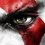# Trigonometry Help

Hey, can anybody provide a solution for this problem? I am totally clueless.

Q.)Find the possible real values of $$(x,y)$$ satisfying $\sin { x } +\cos { x } =\frac { { y }^{ 2 }-2y+4 }{ { y }^{ 2 }+2y+4 }.$Note by Rishi Sharma
5 years, 1 month ago

This discussion board is a place to discuss our Daily Challenges and the math and science related to those challenges. Explanations are more than just a solution — they should explain the steps and thinking strategies that you used to obtain the solution. Comments should further the discussion of math and science.

When posting on Brilliant:

• Use the emojis to react to an explanation, whether you're congratulating a job well done , or just really confused .
• Ask specific questions about the challenge or the steps in somebody's explanation. Well-posed questions can add a lot to the discussion, but posting "I don't understand!" doesn't help anyone.
• Try to contribute something new to the discussion, whether it is an extension, generalization or other idea related to the challenge.

MarkdownAppears as
*italics* or _italics_ italics
**bold** or __bold__ bold
- bulleted- list
• bulleted
• list
1. numbered2. list
1. numbered
2. list
Note: you must add a full line of space before and after lists for them to show up correctly
paragraph 1paragraph 2

paragraph 1

paragraph 2

[example link](https://brilliant.org)example link
> This is a quote
This is a quote
    # I indented these lines
# 4 spaces, and now they show
# up as a code block.

print "hello world"
# I indented these lines
# 4 spaces, and now they show
# up as a code block.

print "hello world"
MathAppears as
Remember to wrap math in $$ ... $$ or $ ... $ to ensure proper formatting.
2 \times 3 $2 \times 3$
2^{34} $2^{34}$
a_{i-1} $a_{i-1}$
\frac{2}{3} $\frac{2}{3}$
\sqrt{2} $\sqrt{2}$
\sum_{i=1}^3 $\sum_{i=1}^3$
\sin \theta $\sin \theta$
\boxed{123} $\boxed{123}$

Sort by:

$\sin x +\cos x \in [-\sqrt{2},\sqrt{2}]$

The range of the right side is $\left[ \frac{1}{3},3 \right]$

So, $\sin x +\cos x ≥\frac{1}{3}$

Now replacing $\sin x =\frac{2t}{1+t^2},\quad \cos x =\frac{1-t^2}{1+t^2}$

Where $t=\tan x$, we will then get,

$2t^2-3t-1≤0 \\ t \in \left[\frac{3-\sqrt{17} }{4}, \frac{3+\sqrt{17}}{4} \right] \\ \therefore x \in \left[ n\pi+\tan^{-1}\left( \frac{3-\sqrt{17}}{4}\right) , n\pi+\tan^{-1}\left( \frac{3+\sqrt{17}}{4}\right)\right]$

- 5 years, 1 month ago

Exactly what @Deeparaj Bhat said... $\sin { x } +\cos { x } =\frac { { y }^{ 2 }-2y+4 }{ { y }^{ 2 }+2y+4 }=k$

$k=\dfrac{y^2-2y+4}{y^2+2y+4}$ $\implies y^2(k-1)-2y(k+1)+4(k-1)=0....(1)$ Since $y\in R$ the discriminate of quadratic must be non-negative. $\implies (k+1)^2-4(k-1)^2\ge 0$ $\implies (3k-1)(k-3)\le 0\implies k\in[\dfrac 13,3]$

While $\sin x+\cos x\in[-\sqrt2,\sqrt 2]$

Hence $\boxed{k\in[\dfrac 13,\sqrt 2]}$

Solve $1$ using quadratic formula:

$\boxed{y=\dfrac { 1+k\pm \sqrt { \left( k-3 \right) \left( 3k-1 \right) } }{ 1-k } },k\ne 1$

While solving $\sin x+\cos x=k$

$\sin \left(x+\dfrac{\pi}{4}\right)=\dfrac k{\sqrt 2}$

$\implies x=-\dfrac{\pi}{4}+2n\pi\pm\sin^{-1}\left(\dfrac{k}{\sqrt 2}\right)$

While when $k=1$, we get $y=0$ while $x=0,\pi/2,2\pi,\dfrac{5\pi}2\cdots$

- 5 years, 1 month ago

Let sinx+cosx=k

It is simple to show that k>= -sqrt(2)

Now, k(y^2+2y+4)=y^2 -2y +4

y^2 (k-1) +2y(k+1) +4(k-1) = 0

For y to be real, D >= 0

This implies 1/3 >= k >= -sqrt(2)

Thus for all real values of x such that k<=1/3 , we will get two real values of y.

I can't solve it further, any help appreciated.

Sorry for no latex.

- 5 years, 1 month ago

- 5 years, 1 month ago

@Akshat Sharda the answer is not correct.

- 5 years, 1 month ago

Can you please elaborate what I've done wrong?

- 5 years, 1 month ago

Even I am confused. First you also need to find the value of y as well. And lastly the correct answer (from a credible source) is $x=2n\pi +\cos { \frac { \pi }{ 4 } \pm } \cos ^{ -1 }{ \frac { k }{ \sqrt { 2 } } } \\ y=\frac { 1+k\pm \sqrt { \left( k-3 \right) \left( 3k-1 \right) } }{ 1-k } \quad ;\quad k\in \left[ \frac { 1 }{ 3 } ,\sqrt { 2 } \right] -\left\{ 1 \right\}$

- 5 years, 1 month ago

In the expression for $x$, it should've been $\frac{\pi}{4}$ instead of its cosine and for $k=1$, we have $y=0$.

Now, coming to how to solve it. Put the RHS as $k$. Note that $k \leq \sqrt{2}$ due to the LHS.

Also, via calculus (or inequalities) you can see that $\frac{1}{3} \leq k$ (as $y$ is real).

Now, finding $x$ in terms of $k$ is easy.

Also, for $k \neq 1$ we can solve for $y$ as a quadratic. If $k=1$, then $y=0$.

And we're done.

- 5 years, 1 month ago

Thanks. Got it

- 5 years, 1 month ago